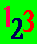The ABC Study Guide, University education in plain English alphabetically indexed. Click here to go to the main index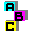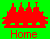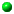Figure Home PageTips for the anxious

# ABC Mathematics

#### Words to help you think about numbers and arithmetic Space, shape and geometry algebra statistics other aspects of mathematics

Mathematical thinking is important for everyone. It is as important as any thinking that we do with just words. It is also something that we often do without realising we do it.

In his History of the Mathematical Sciences, Ivor Grattan-Guinness speculates about the intuitive mathematical thinking that would have been necessary for early humans.

He thinks that early humans did not use any thinking with elements of statistics, algebra or calculus, but that their intuitive thinking did include counting and sorting (which lead to arithmetic); spacing and distancing (which lead to geometry); balancing and weighing (which lead to statics); moving and hitting (which lead to dynamics); guessing and judging (which lead to probability theory) and collecting and ordering (which led to part-whole theory). ( Grattan-Guinness, I. 1997 p.19)

This part of the study guide tries to explain mathematical ideas in plain English and to link these explanations to the other parts of the Study Guide, such as the science time line, the general words we use for thinking, and the advice about research.

A number is a word or symbol used for counting or to say where something comes in a series.

Counting is either

• saying all the numbers in order, up to a certain number
• adding the number of things to see how many there are.

Different Kinds of Numbers

Natural Numbers: Natural numbers are the numbers we use for counting, and for calculating. There is an endless chain of these numbers, usually starting with one: 1, 2, 3, 4, 5, 6, etc. Sometimes zero (0) is included.

Integers: are the natural numbers plus their negatives, including zero. Whilst the natural numbers form an endless chain going upwards from zero, the integers form an endless chain going up and down from zero:
<<< -6, -5, -4, -3, -2, -1, 0, 1, 2, 3, 4, 5, 6, >>>

Cardinal Numbers: Cardinal numbers are numbers we use for counting. Any number that shows us how many there are (e.g. two, forty, 627) is a Cardinal Number. Cardinal numbers do not tell us anything about the order of the objects being counted. That is the job of Ordinal Numbers.

Cardinal numbers are numbers we use for counting, but not for calculating. They are the natural numbers used only for counting. If you count the buttons on your jacket, you are using cardinal numbers. If you count the buttons on your jacket and then add five, you are no longer using cardinal numbers, because you are calculating.

Ordinal Numbers: Ordinal numbers are used to show the places in a sequence: 1st, 2nd, 3rd, 4th, 5th, 6th, etc.

Rational Numbers: Rational numbers include
integers and fractions, but not those numbers, like the square root of two, that we cannot state as an exact fraction.

Real Numbers: Real numbers are the
Rational Numbers plus those numbers (like the square root of two) that we cannot state as an exact fraction, as long as we can place them in a series. The Real Numbers are the numbers used in measurement. The square root of two centimetres could not be expressed as an exact fraction, but you could show where it is on a straight line.

"Pi is the ratio of the circumference of a circle to its diameter... it is an irrational number that cannot be expressed as the ratio of two whole numbers and has an apparently random decimal string of infinite length" 3.14159265......... for ever   (external link)

Complex Numbers: Complex Numbers are Real Numbers plus numbers called Imaginary Numbers that cannot be placed in a series. The square root of minus one is an imaginary number. It is not a "real" number, but it is real enough to be used in an equation that describes the behaviour of an alternating current of electricity.

Some of the different kinds of numbers we use are related to the different kinds of scales we use to classify, quantify, and measure things.

An attribute is a property. Redness, being on and being a baby, are all attributes. Something either has or does not have a particular attribute. As a scale there are only two positions: yes, the attribute is present; or no, the attribute is not present. "Binary" means having two possibilities. The convention for binary coding is to use 1 where the attribute is present (something is red, for example) and 0 when it is absent.

Nominal Scale

In a nominal scale, names (which may be numbers) are given to things just to label and classify them. Dividing people into male and female is a nominal scale. Putting numbers on buses to identify the route is a nominal scale. Putting numbers on football players to identify their position on the field is a nominal scale.

Arithmetical operations are meaningless on nominal scales. To add a 44 bus to a 102 bus and get a 146 bus is nonsense. But if data has been classified nominally you can make a Frequency Distribution - you can count how many 102 buses and how many 146 buses there are, for example.

The ordinal scale puts things in an order or rank, as well as labelling and classifying them. House numbers are usually on an ordinal scale because 1 is usually at the beginning of the road and the higher the number the further down the road it is.

An interval scale puts the items at an equal distance from one another (the interval), as well as ranking them in order. Years are counted on interval scales. There is the same distance between one year and its neighbours as there is between any other year and its neighbours. 1997 is the same distance from 1996 and 1998 as 1953 was from 1952 and 1954.

Interval scales do not have "absolute zeros" or real starting points. The year 1996 is numbered from the birth of Christ (which is labelled 1), but there are years before that.

We can use real numbers to add to, subtract from, multiply and divide by items on the interval scale. The year 1996 divided by 2 is the year 998. This is not "half" of the year 1996, however, because an interval scale does not have an absolute zero.

But we can take two numbers on the scale and divide by two (average) to find out the date that is half way between the two. 998 is half way between the year 10 and the year 1986 because 10 plus 1986 = 1996, which divided by two equals 998. Similarly, we can take any other arithmetic mean: The average of 1991, 1993, 1997 and 1998 is (1991 + 1993 + 1997 + 1998) divided by four, which equals = 1994.75 (the autumn of 1994).

Ratio Scale

Ratio scales have an absolute zero as well as equal intervals between the items. A tape measure is an example of a ratio scale. Zero (0) on the tape measure really is nothing. That is where the measuring starts. Having a true zero also means (by implication) that there is a range of negative numbers possible.

With a ratio scale we can say that one point on the scale is so much more or less than another. 10 centimetres is twice 5 centimetres and half of 20 centimetres. We cannot do this with simple interval scales. The year 1998 is not twice a previous year or half of a future year.

Ratio scales are real numbers. They have order, equal intervals and an absolute zero. It is because real numbers have these characteristics that we can perform the operations of mathematics with them. Real numbers and ratio scales can be added, subtracted, multiplied and divided. They can also be raised to powers or brought down to roots, and this means that we can take logarithms and use calculus with them.

A series is a number of similar or related events or things, one following another. The word comes from the Latin for a row, chain (or series), based on the Latin for join or connect. In English, the word developed in the 17th century. Before that, people would have talked of a chain (a number of things linked to one another in a line) or train (where a number of things are pulled forward by the first).

Scale can have two meanings. Two bowls hung on a piece of string can be used to find out which of two objects is the heaviest. These are scales as in the "scales of justice". A ladder or stairs has a series of steps at equal intervals, these are like the marks on a ruler that enable us to measure the "scale" (size) of an object and which are also like "musical scales". The first idea of scale comes from germanic words for bowls and cups. The second comes from the Latin word for staircase or ladder - and so goes back to the idea of walking, where your steps have an equal length.

See:Different Kinds of Scale (like binary scale and nominal scale)Progression

See Maths is Fun's most common mathematical symbols

See Wikipedia's list of mathematical symbols

Includes Σ

Special Numbers

Zero and the arabic number system

Zero is the number 0 (nothing). It is one of the great discoveries of mathematics made in India. It made possible a system of numbers that we call "arabic", because it came to Western Europe through arab mathematicians.

To understand this revolution in mathematics, you will first need to look at some of the problem of the Roman system of numbers that Western Europe used before we adopted arabic numbers.

Roman numerals are still used occasionally. The BBC, for example, uses them to show the year its programmes were made in.

The Romans used letters for numbers.

I is one.

To make two you put two I s together: II

To make three you put three I s together: III .

To stop the numbers becoming very long they used other letters for some larger numbers and made the numbers between the letters by taking letters away or adding them.

V is five.

To make four they put I in front of V to indicate that the one should be taken away from five: IV .

To make six they put I after V to indicate that the one should be added to five: VI .

The Romans used
I for one,
V for 5,
X for ten,
L for 50,
C for 100,
D for 500 and
M for a thousand.

The same principles of adding and taking away letters are used throughout, so

MM is 2,000,
MD is 1,500,
XX is 20,
XXIV is 24.

If you have tried to understand the dates of BBC productions, you will know that most Roman numbers take time to decipher.

MCMXCVII , for example, is 1997.

The M is 1,000,
to this is added CM for 900,
then XC for 90,
then VII for seven.

The difficulty gets much worse if you want to do arithmetic in Roman numerals. How, for example, do you work out how many years before 2000 the year 1997 was in Roman numerals?

 This is how it can be written: MM take away MCMXCVII is III Eric kindly corrected my Roman arithmetic. When anyone finds errors, please tell me!

But how would you work the answer out? If I find out, I will tell you. I suspect that you need a special instrument, like an abacus (a counting machine made of beads on wires). [See article by Steve Stephenson about The Roman Hand-Abacus and timeline on the Roman Empire]

You can use an electronic calculator on the world wide web to convert roman to arabic numbers and the other way round: See Nova Romana: Roman Numbers

The Arabic system can also be called the Hindu or Indian system.

The Arabic system has ten digits: 0, 1, 2, 3, 4, 5, 6, 7, 8, 9

The Roman system had no sign for zero because counting nothing can be done by not doing it. The arabic system uses the same digits in different positions to indicate different multiples of ten. It needs a mark for nothing to show position when the position slot is empty. For example:

```  1 is one
10 is ten
100 is one hundred
```
This system makes arithmetic much easier. For example, when work out how many years before 2000 the year 1997 was in arabic numerals, we write it down like this:

 2000 1997 3

The calculation begins on the right of the numbers and moves to the left (the opposite direction to the one we read in, because the smallest number is on the right).

The first 0 in 2000 is the ten position. A 7 is immediately beneath it, so take 7 from 10 = 3. We write that down.

When we treat 0 as ten (10 - 7 =3) instead of as nothing (0 - 7 = -3) we carry the 1 from the ten along to the next digit on the second line and add it.

9 + 1 = 10, so taking the second line from the first in the second position is 0 - 0 = 0

The same happens with the third position.

For the fourth position we are carrying one forward, so 1 becomes 2 and 2 - 2 = 0.

Most people will be so used to doing this that thinking about what they actually do will be quite difficult. A little reflection, however, should convince you that the arabic system is much easier than the Roman. It is also much more powerful.

Formulas

A =r ²

is the formula to find out the area of a circle. To use it you have to understand the symbols, remember the rule and know when to apply it.

A is areais a Greek letter (pi) used to indicate a number which no one has been able to calculate in full, but which begins: 3.14159265

If we write a formula as simple steps, it becomes an algorithm

This is an algorithm for the area of a circle:

Multiply the result by an approximation to pi

Write down the answer as the area of the circle

Mathematics

The study of ideas like number, quantity, arrangement, shape and space.

The parts of mathematics include

Model See the Wikipedia article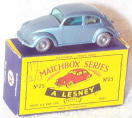Why is a car that fits in a matchbox a model car? Why is the student who can answer these questions a model student?

Mathematical Models

Mathematics provides us with models of external reality. One can think of the models as being

• thought structures based on external reality ( empiricism) or
• thought structures applied to external reality ( rationalism).
Pure mathematics has no necessary relation to external reality, it is a thought form. Applied mathematics uses some of these thought forms as models of external reality.

A model has a clearer shape than the real thing. It does not have all the confusing detail of the external reality and it allows us to manipulate an idea of the reality in our minds.

Example:

You are travelling on a train when a sign "London 100 miles" flashes by the window. Looking at your watch you calculate that the train is due in London in two hours. You think that means the train is only travelling at fifty miles an hour.

In reality the speed of the train varies considerably on the journey. Sometimes it stands still at stations and sometimes it goes much faster than fifty miles an hour.

It is only by using a simplified model of complex reality that you are able to make calculations about the speed of the train and when it will arrive at different stations.

(Based on Open University DDB)

Catchup Mathematics

Numeracy
The word numeracy is used, by different people, to cover a different ranges of skill related to mathematics. Here are some definitions:Study Link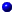Andrew Roberts' web Study GuideTop of PageTake a Break - Read a PoemClick coloured words to go where you want

© Andrew Roberts 7.1999 - 3.2001

#### Mathematics index

*****************

binary = using two
Bi. Arithmetic
Bi. Calculator
Binary Number
Binary Scale

cent = hundredth
centigram
centilitre
centimetre
century

deca = ten times
decagram
decalitre
decametre

deci = tenth
decigram
decilitre
decimetre

giga (giant)
= 1,000 million
gigabyte

Gram
Graph

hecto = 100 times
hectogram
hectolitre
hectometre
Hertz

kilo = 1,000 times
kilobyte
kilogram
kilolitre
kilometre

mega (great)
= million
megabyte
Megahertz

Metre

metric = using ten
Metric

milli = thousandth
milligram
millilitre
millimetre

*****************

This index
was suggested by
Melissa Claydon

*****************

 Quapropter bono christiano, sive mathematici, sive quilibet impie divinantium, maxime dicentes vera, cavendi sunt, ne consortio daemoniorum irretiant [Augustine] has recently been translated as "Hence, a devout Christian must avoid astrologers and all impious soothsayers, especially when they tell the truth, for fear of leading his soul into error by consorting with demons and entangling himself with the bonds of such association" (see external link) Mathematicus is mathematical, but mathematici can be mathematician or astrologer.
